# Lyapunov characteristic exponent

(diff) ← Older revision | Latest revision (diff) | Newer revision → (diff)

of a solution of a linear system

The limes superior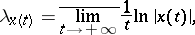whereis a solution of the linear system of ordinary differential equations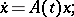(1)

here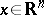and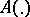is a mapping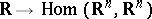, summable on every interval. In coordinates,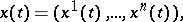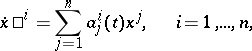where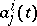are functions summable on every interval and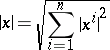(or any other equivalent norm;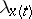does not depend on the choice of the norm inor in).

Lyapunov's theorem. Suppose that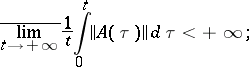equivalently: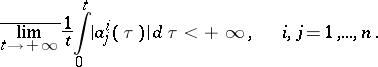Then for any solution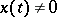of the system (1) the Lyapunov characteristic exponentis a real number (that is,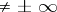). The following assertions hold for the Lyapunov characteristic exponents of non-zero solutions of (1):

1),;

2)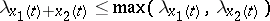;

3) there exists a set of linearly independent solutions of (1), denoted by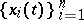, such that for any otherlinearly independent solutions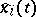,, of (1), numbered in decreasing order of the Lyapunov characteristic exponents, that is,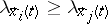for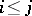, the following inequalities hold:A fundamental system of solutionswith this property is called normal. Such a normal system has the properties:

a) the family of numbers,, does not depend on the choice of the normal fundamental system;

b) for any solutionof (1) its Lyapunov characteristic exponentis equal to some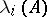;

c),.

The numbers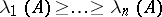are called the Lyapunov characteristic exponents of the system (1); the number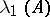is often called the leading Lyapunov characteristic exponent of (1).

The set of all Lyapunov characteristic exponents of non-zero solutions of (1) is called the spectrum.

## Contents

### Special case.

1) A system with constant coefficients (that is,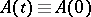). In this case theare equal to the real parts of the eigen values of the operator(the matrix).

2) A system with periodic coefficients (that is,,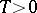). In this case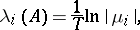where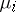are the multipliers of the system (1), numbered in non-increasing order of their moduli (each is taken as many times as its multiplicity).

The role of the Lyapunov characteristic exponent in the theory of Lyapunov stability is based on the following assertion: If(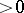), then the solutions of (1) are asymptotically stable (respectively, unstable, cf. Asymptotically-stable solution). Fromit does not follow that the null solution of the system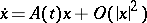is Lyapunov stable; however, if it is also known that the system (1) is a regular linear system, then this conclusion is valid (Lyapunov's theorem).

Suppose that the systemis obtained by a small perturbation of a system (1) satisfying the condition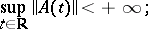that is, the distance between them, defined by the formula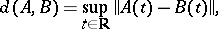(2)

is small. Forthis does not imply that the quantity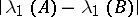is small (it is implied if the system (1) has constant or periodic coefficients, and also for certain other systems); in other words, the functionalsare not everywhere continuous on the space of systems (1) (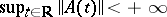), endowed with the given metric (2).

Lyapunov characteristic exponents were introduced by A.M. Lyapunov, not only for solutions of the system (1), but also for arbitrary functions on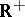(see ).

How to Cite This Entry:
Lyapunov characteristic exponent. Encyclopedia of Mathematics. URL: http://encyclopediaofmath.org/index.php?title=Lyapunov_characteristic_exponent&oldid=12994
This article was adapted from an original article by V.M. Millionshchikov (originator), which appeared in Encyclopedia of Mathematics - ISBN 1402006098. See original article